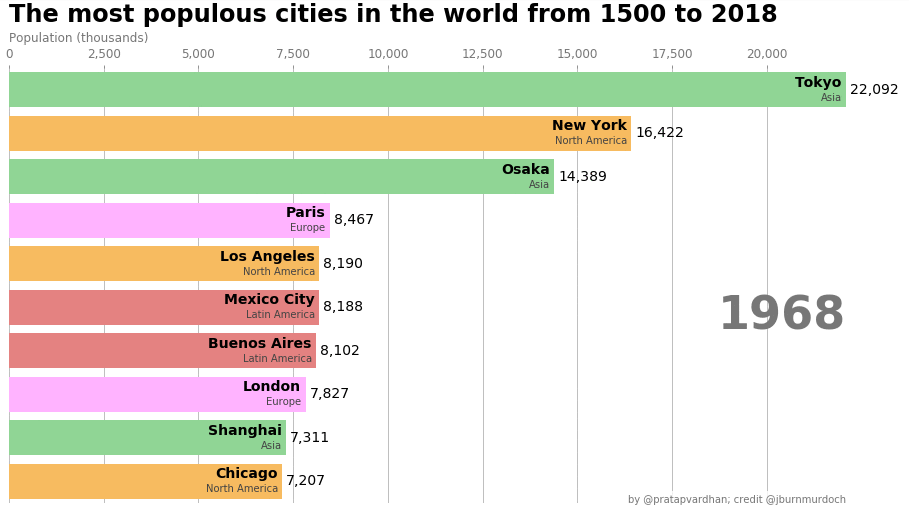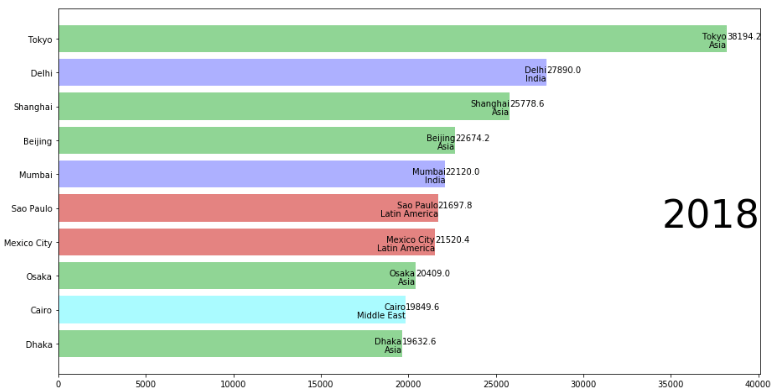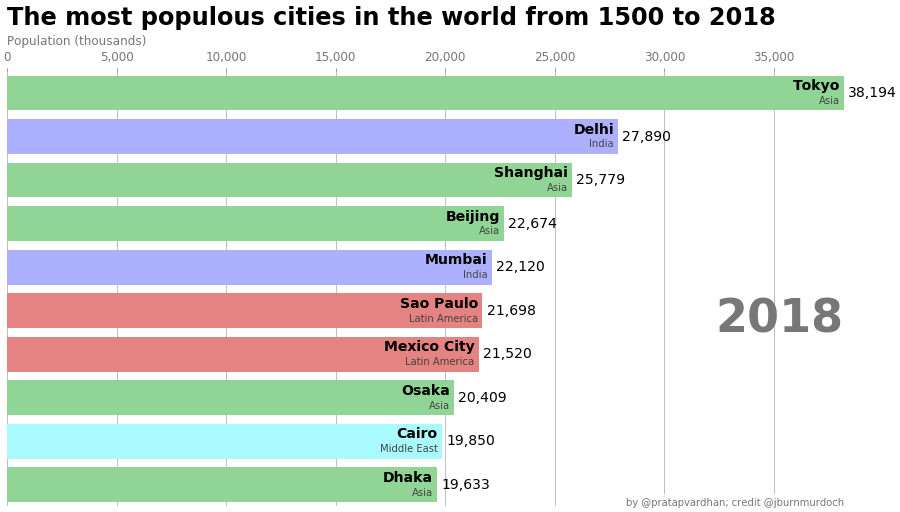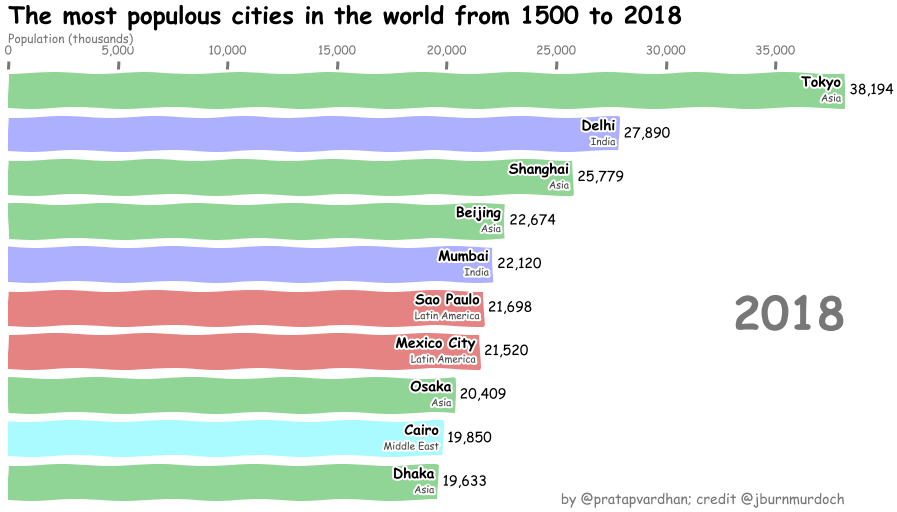Bar Chart Race in Python with Matplotlib
04. Sep 2019

~In roughly less than 50 lines of code

Republished on towardsdatascience

Bar chart races have been around for a while. This year, they took social media by storm. It began with Matt Navarra’s tweet, which was viewed 10 million times. Then, John Burn-Murdoch created reproducible notebook using d3.js, others started creating their races. Then, Flourish studio released race chart for non-programmers. Since then hundreds of races have been shared on the Internet.

## Race with Matplotlib

I wondered – How easy would it be to re-produce JBM’s version in Python using Jupyter and Matplotlib? Turns out, in less than 50 lines of code, you can reasonably re-create reusable bar chart race in Python with Matplotlib.

Here’s what we want to create.## Let’s Code

Now, that you’ve seen the output, we’ll incrementally build it up.

`Matplotlib`'s style defaults are designed for many common situations, but are in no way optimal for our aesthetics. So, bulk of our code would go into styling (axes, text, colors, ticks etc)

In your `Jupyter` notebook, import the dependent libraries.

``````import pandas as pd
import matplotlib.pyplot as plt
import matplotlib.ticker as ticker
import matplotlib.animation as animation
from IPython.display import HTML
``````

Read the city populations dataset with `pandas`. We only need 4 columns to work with `'name', 'group', 'year', 'value'`. Typically, a `name` is mapped to a `group` (city to continent/country) and each `year` has one `value`.

``````df = pd.read_csv('https://git.io/fjpo3', usecols=['name', 'group', 'year', 'value'])
``````
name group year value
0AgraIndia1575200.0
1AgraIndia1576212.0
2AgraIndia1577224.0

## Data transformations

We are interested to see top 10 `value`s are a given `year`. Using pandas transformations, we will get top `10` values.

``````current_year = 2018
dff
``````
name group year value
6045 TokyoAsia201838194.2
1324 DelhiIndia201827890.0
5547 ShanghaiAsia201825778.6
689 BeijingAsia201822674.2
3748 MumbaiIndia201822120.0
5445 Sao PauloLatin America201821697.8
3574 Mexico CityLatin America201821520.4
4679 OsakaAsia201820409.0
1195 CairoMiddle East201819849.6
1336 DhakaAsia201819632.6

## Basic chart

Now, let’s plot a basic bar chart. We start by creating a figure and an axes. Then, we use `ax.barh(x, y)` to draw horizontal barchart.

``````fig, ax = plt.subplots(figsize=(15, 8))
ax.barh(dff['name'], dff['value'])
``````Notice, highest bar is at the bottom. We need to flip that.

## Color, Labels

Next, let’s add values, group labels and colors based on groups. We’ll user `colors` and `group_lk` to add color to the bars.

``````colors = dict(zip(
['India','Europe','Asia','Latin America','Middle East','North America','Africa'],
['#adb0ff', '#ffb3ff', '#90d595', '#e48381', '#aafbff', '#f7bb5f', '#eafb50']
))
group_lk = df.set_index('name')['group'].to_dict()
``````

`group_lk` is mapping between `name` and `group` values.

``````fig, ax = plt.subplots(figsize=(15, 8))
dff = dff[::-1]   # flip values from top to bottom

# pass colors values to `color=`

ax.barh(dff['name'], dff['value'], color=[colors[group_lk[x]] for x in dff['name']])
# iterate over the values to plot labels and values (Tokyo, Asia, 38194.2)

for i, (value, name) in enumerate(zip(dff['value'], dff['name'])):
ax.text(value, i,     name,            ha='right')  # Tokyo: name

ax.text(value, i-.25, group_lk[name],  ha='right')  # Asia: group name

ax.text(value, i,     value,           ha='left')   # 38194.2: value

# Add year right middle portion of canvas

ax.text(1, 0.4, current_year, transform=ax.transAxes, size=46, ha='right')
``````Now, we’re left with styling the chart.

## Polish Style

For convenience let’s move our code to `draw_barchart` function.

We need to style following items:

• Text: Update font sizes, color, orientation
• Axis: Move X-axis to top, add color & subtitle
• Grid: Add lines behind bars
• Format: comma separated values and axes tickers
• Add title, credits, gutter space
• Remove: box frame, y-axis labels

We’ll add another dozen lines of code for this.

``````fig, ax = plt.subplots(figsize=(15, 8))

def draw_barchart(year):
dff = df[df['year'].eq(year)].sort_values(by='value', ascending=True).tail(10)
ax.clear()
ax.barh(dff['name'], dff['value'], color=[colors[group_lk[x]] for x in dff['name']])
dx = dff['value'].max() / 200
for i, (value, name) in enumerate(zip(dff['value'], dff['name'])):
ax.text(value-dx, i,     name,           size=14, weight=600, ha='right', va='bottom')
ax.text(value-dx, i-.25, group_lk[name], size=10, color='#444444', ha='right', va='baseline')
ax.text(value+dx, i,     f'{value:,.0f}',  size=14, ha='left',  va='center')
# ... polished styles

ax.text(1, 0.4, year, transform=ax.transAxes, color='#777777', size=46, ha='right', weight=800)
ax.text(0, 1.06, 'Population (thousands)', transform=ax.transAxes, size=12, color='#777777')
ax.xaxis.set_major_formatter(ticker.StrMethodFormatter('{x:,.0f}'))
ax.xaxis.set_ticks_position('top')
ax.tick_params(axis='x', colors='#777777', labelsize=12)
ax.set_yticks([])
ax.margins(0, 0.01)
ax.grid(which='major', axis='x', linestyle='-')
ax.set_axisbelow(True)
ax.text(0, 1.12, 'The most populous cities in the world from 1500 to 2018',
transform=ax.transAxes, size=24, weight=600, ha='left')
ax.text(1, 0, 'by @pratapvardhan; credit @jburnmurdoch', transform=ax.transAxes, ha='right',
color='#777777', bbox=dict(facecolor='white', alpha=0.8, edgecolor='white'))
plt.box(False)

draw_barchart(2018)
``````We now have an identical chart.

## Animate Race

To animate the race, we will use `FuncAnimation` from `matplotlib.animation`. `FuncAnimation` makes an animation by repeatedly calling a function (that draws on canvas). In our case, that function will be `draw_barchart`. We also use `frames`, this argument accepts on what values you want to run `draw_barchart` – we’ll run from `year` 1968 to 2018.

``````import matplotlib.animation as animation
from IPython.display import HTML

fig, ax = plt.subplots(figsize=(15, 8))
animator = animation.FuncAnimation(fig, draw_barchart, frames=range(1968, 2019))
HTML(animator.to_jshtml())
# or use animator.to_html5_video() or animator.save()

``````

And, there, we have it, bar chart race inside a notebook with matplotlib.You could save the `animator` object to a video/gif or play within the notebook.

## Bonus: xkcd-style!

Turning your matplotlib plots into xkcd styled ones is pretty easy. You can simply turn on `xkcd` sketch-style drawing mode with `plt.xkcd`.

``````with plt.xkcd():
fig, ax = plt.subplots(figsize=(15, 8))
draw_barchart(2018)
``````Here’s the animated xkcd-styled bar chart race.

## DIY

Full code for the race animation is here and you can play with it on Google Colab also. Try changing the dataset, colors and share your races.

Matplotlib is a massive library – being able to adjust every aspect of a plot is powerful but it can be complex / time-consuming for highly customized charts. Atleast, for these bar chart races, it was fairly quick!